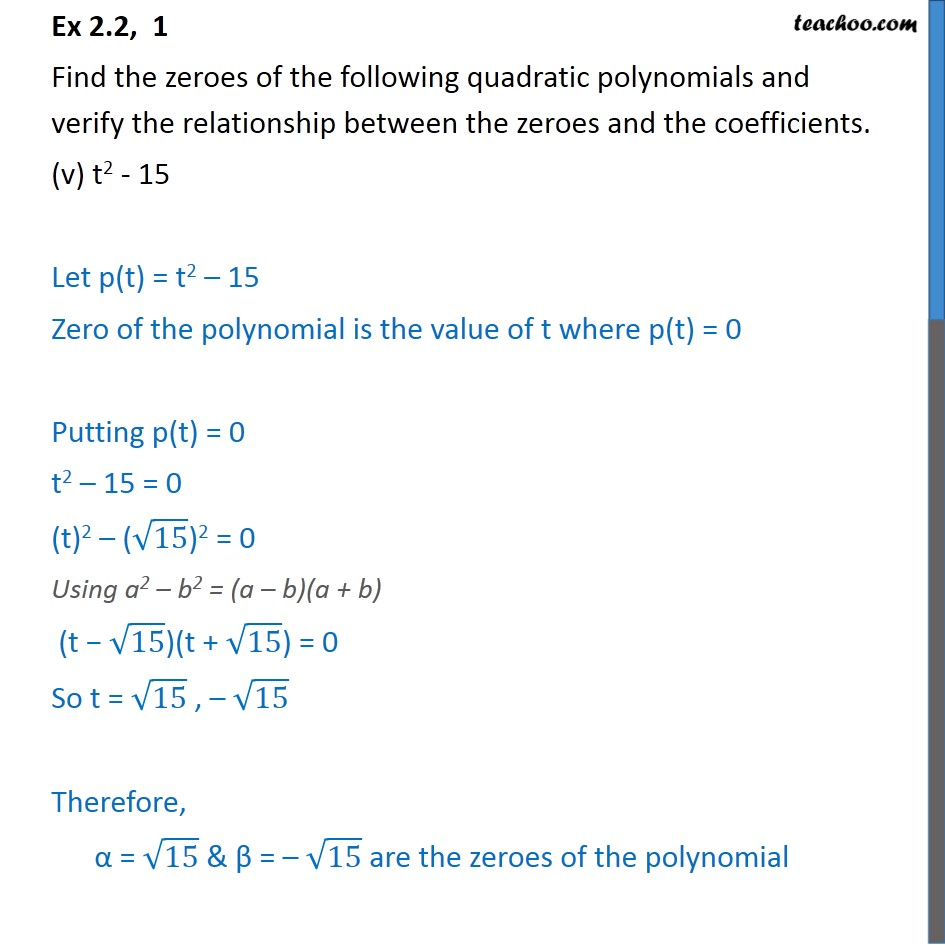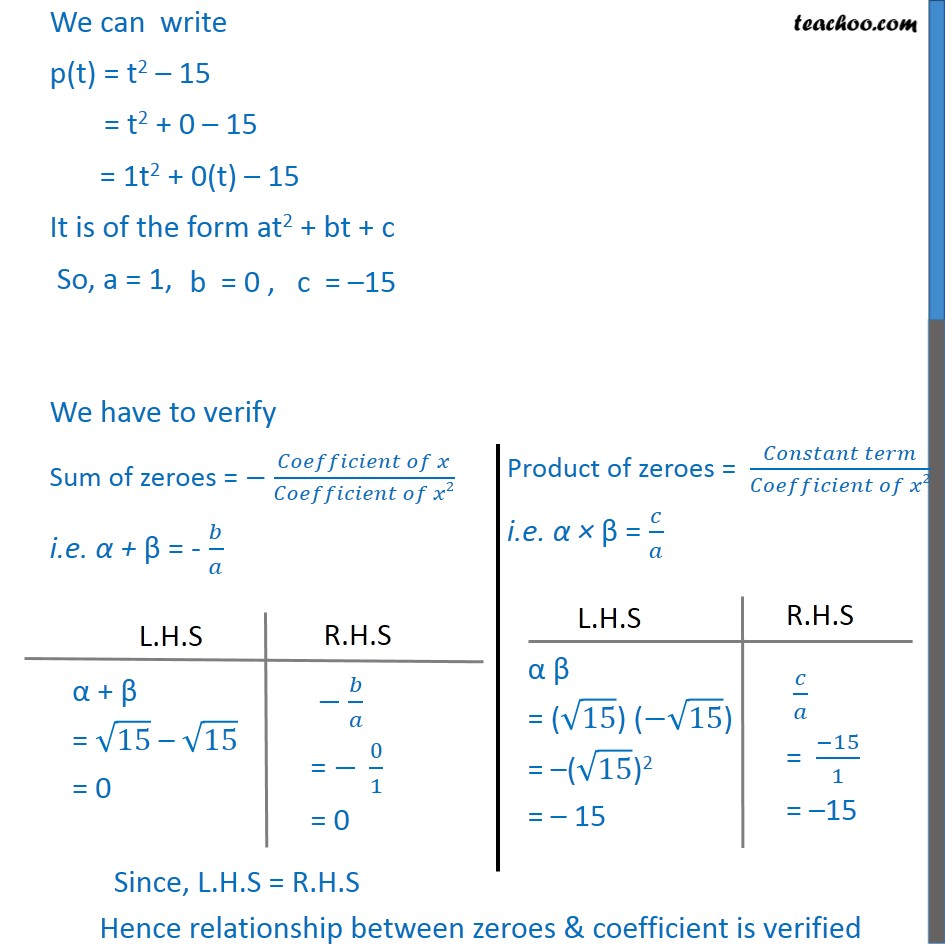1. Chapter 2 Class 10 Polynomials (Term 1)
2. Serial order wise
3. Ex 2.2

Transcript

Ex 2.2, 1 Find the zeroes of the following quadratic polynomials and verify the relationship between the zeroes and the coefficients. (v) t2 - 15 Let p(t) = t2 – 15 Zero of the polynomial is the value of t where p(t) = 0 Putting p(t) = 0 t2 – 15 = 0 (t)2 – (√15)2 = 0 Using a2 – b2 = (a – b)(a + b) (t − √15)(t + √15) = 0 So t = √15 , – √15 Therefore, α = √15 & β = – √15 are the zeroes of the polynomial We can write p(t) = t2 – 15 = t2 + 0 – 15 = 1t2 + 0(t) – 15 It is of the form at2 + bt + c So, a = 1, We have to verify Sum of zeroes = − (𝐶𝑜𝑒𝑓𝑓𝑖𝑐𝑖𝑒𝑛𝑡 𝑜𝑓 𝑥)/(𝐶𝑜𝑒𝑓𝑓𝑖𝑐𝑖𝑒𝑛𝑡 𝑜𝑓 𝑥2) i.e. α + β = - 𝑏/𝑎